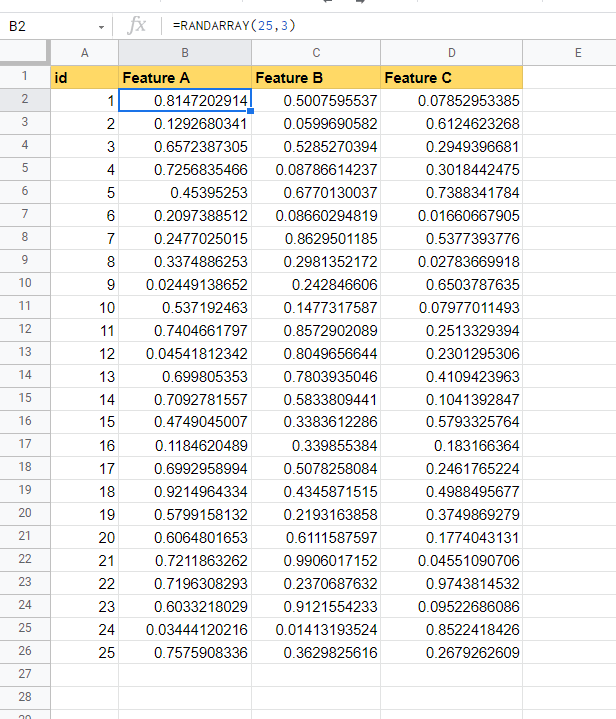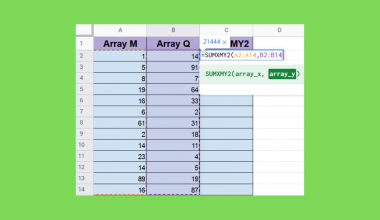How To Use RANDARRAY Function in Google Sheets

The RANDARRAY function in Google Sheets is useful when you need to generate an array of random numbers between 0 and 1.

You can specify the size of the array by specifying the number of rows and columns the array will contain.

The rules for using the RANDARRAY function in Google Sheets are as follows:

• The function requires two arguments: the number of rows and columns the array of random number needs
• The function then outputs an array of random numbers between 0 and 1 at a specified size
• Hitting the Enter key again will refresh the function and update the random numbers.

For example, we would like to create a sample dataset. We know that we can use the RAND() function to output a number between 0 and 1. However, if we need multiple columns filled with random values, we would have to use RAND to generate each cell. Luckily, with the RANDARRAY function, it becomes simple to generate a table of any size filled with random data.

We have now seen one possible use-case for the RANDARRAY function. Let’s go deeper into how we can actually use it and later work on an actual sample spreadsheet.

The Anatomy of the RANDARRAY Function

The syntax of the RANDARRAY function is as follows:

=ROUNDARRAY(rows, columns)

Let’s break apart this formula and understand what each of these terms means:

• = the equal sign is how we start any function in Google Sheets.
• RANDARRAY() is our RANDARRAY function. It generates an array of random numbers from 0 to 1.
• rows refers to how many rows the array generated should contain.
• columns indicate how many columns the array generated should have
• If rows and columns are not specified, RANDARRAY returns a 1 x 1 sized grid.

A Real Example of Using RANDARRAY Function

Let’s take a look at an example of the RANDARRAY function being used in a Google Sheets spreadsheet.In the table above, we were able to use the RANDARRAY function to fill up three columns with 25 rows each. The values of these cells will update if you re-enter the formula.

To get the generated values, we just need to use the following formula in cell B2:

=RANDARRAY(25,3)

In this next example, we were able to fill another dataset with a differently sized array.You can make your own copy of the spreadsheet above using the link I have attached below.

If you’re ready to try the RANDARRAY function yourself in Google Sheets, let’s begin writing it ourselves!

How to Use RANDARRAY Function in Google Sheets

1. To start using the RANDARRAY function, look for the location which will be the upper-left most cell in the array. In this case, we can write our formula in cell B2.2. Next, we just have to type the equal sign ‘=‘ to begin the function, followed by ‘RANDARRAY(‘.
3. A tooltip box appears with info on the RANDARRAY function. We can click on the arrow on the top-right-hand corner of the box to minimize it if necessary.4. Next, we need to specify the number of rows and columns the output array should have. In this case, we need 25 rows and 3 columns in our array.
Afterward, simply hit Enter on your keyboard to let the function evaluate.5. If you would like to keep the values from re-updating, you can copy the values over. To do this, first select the array and type Ctrl + C to copy the selected array. Next, you can type Ctrl + Shift + V to paste only the values of the copied array.
Alternatively, you may right-click on the selection and select Paste Special > Values only from the menu. After doing so, your randomly generated values will no longer update after every refresh.1. Can I use RANDARRAY to create an array of random integers between arbitrary values?
Though the output of RANDARRAY are values between 0 and one, we can use other functions to expand functionality.
In the example below, we’ve used an alternate formula to set a minimum and maximum range from which to choose numbers from. We’ve also used the INT function to make sure that all of the numbers in the array are integers.2. Why does my formula return a #NUM! error?
The arguments of your RANDARRAY function must be a positive number. If the number is negative, the function will return a #NUM! error instead.

This step-by-step guide shows how easy it is to generate an array of random values from 0 to 1.  Hopefully, you now have an idea of when to use the RANDARRAY function after seeing multiple examples of it in action.

You can now use the RANDARRAY functions in Google Sheets together with the various other Google Sheets formulas available to create great worksheets that solve your problems.

Are you always looking for new ways to use Google Sheets? You can find more helpful tips and tricks like this by subscribing to our newsletterOur goal this year is to create lots of rich, bite-sized tutorials for Google Sheets users like you. If you liked this one, you'd love what we are working on! Readers receive ✨ early access ✨ to new content.

You May Also LikeHow to Find the Last Matching Value in Google Sheets

To find the last matching value in Google Sheets is useful if you want to find the last…How to Use MAX Function in Google Sheets

The MAX function in Google Sheets is useful when you need to get the maximum value in a…How to Use TO TEXT Function in Google Sheets

The TO_TEXT function in Google Sheets turns a number into a text.  The function helps you easily convert…How to Use SUMXMY2 Function in Google Sheets

The SUMXMY2 function in Google Sheets is a mathematical function designed specifically to return the sum of squares…